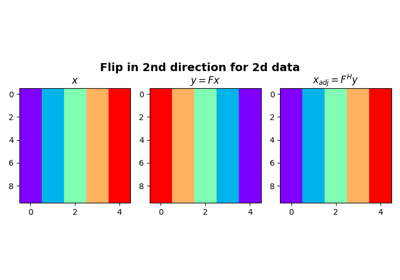# pylops.Flip#

class pylops.Flip(dims, axis=-1, dtype='float64', name='F')[source]#

Flip along an axis.

Flip a multi-dimensional array along axis.

Parameters
dimslist or int

Number of samples for each dimension

axisint, optional

New in version 2.0.0.

Axis along which model is flipped.

dtypestr, optional

Type of elements in input array.

namestr, optional

New in version 2.0.0.

Name of operator (to be used by pylops.utils.describe.describe)

Notes

The Flip operator flips the input model (and data) along any chosen direction. For simplicity, given a one dimensional array, in forward mode this is equivalent to:

$y[i] = x[N-1-i] \quad \forall i=0,1,2,\ldots,N-1$

where $$N$$ is the dimension of the input model along axis. As this operator is self-adjoint, $$x$$ and $$y$$ in the equation above are simply swapped in adjoint mode.

Attributes
shapetuple

Operator shape

explicitbool

Operator contains a matrix that can be solved explicitly (True) or not (False)

Methods

 __init__(dims[, axis, dtype, name]) adjoint() apply_columns(cols) Apply subset of columns of operator cond([uselobpcg]) Condition number of linear operator. conj() Complex conjugate operator div(y[, niter, densesolver]) Solve the linear problem $$\mathbf{y}=\mathbf{A}\mathbf{x}$$. dot(x) Matrix-matrix or matrix-vector multiplication. eigs([neigs, symmetric, niter, uselobpcg]) Most significant eigenvalues of linear operator. matmat(X) Matrix-matrix multiplication. matvec(x) Matrix-vector multiplication. reset_count() Reset counters rmatmat(X) Matrix-matrix multiplication. rmatvec(x) Adjoint matrix-vector multiplication. todense([backend]) Return dense matrix. toimag([forw, adj]) Imag operator toreal([forw, adj]) Real operator tosparse() Return sparse matrix. trace([neval, method, backend]) Trace of linear operator. transpose()

## Examples using pylops.Flip#Flip along an axis

Flip along an axis